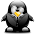## Wednesday, September 23, 2015

### Memory Layout of a C program

A typical memory representation of C program consists of following sections.

1. Text segment
2. Initialized data segment
3. Uninitialized data segment
4. Stack
5. HeapLet us consider a general program to understand this concept.

`#include <stdio.h>`
`int` `global1 = 20; ``/* initialized global variable stored in DS*/`
`int global2;    /* Uninitialized global variables stored in BSS segment*/`
`int global3=0;  /* Global variables initialized to zero are stored in BSS segment*/`
`int` `main(``void``)`
`{`
`    ``static` `int` `i = 100; ``/* Initialized static variable stored in DS*/`
`    static int j=0;    /* Static variables initialized to 0 are stored in BSS segment*/ `
`    int l=100;  /* stack stores the auto variables*/       `
`    int k; ``     /* stack stores the auto variables*/    `
`return` `0;`
`}`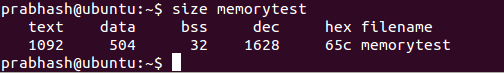Let us now comment an auto local variable

`#include <stdio.h>`
`int` `global1 = 20; ``/* initialized global variable stored in DS*/`
`int global2;    /* Uninitialized global variables stored in BSS segment*/`
`int global3=0;  /* Global variables initialized to zero are stored in BSS segment*/`
`int` `main(``void``)`
`{`
`    ``static` `int` `i = 100; ``/* Initialized static variable stored in DS*/`
`  static int j=0;    /* Static variables initialized to 0 are stored in BSS segment*/ `
`    //int l=100;  /* stack stores the auto variables*/       `
`    int k; ``     /* stack stores the auto variables*/    `
`return` `0;`
`}`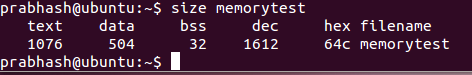As the local variables reside in stack so the size of data as well as bss segement remained the same.

`Let us now comment an intialized global variable ,global1`

`#include <stdio.h>`
`//int` `global1 = 20; ``/* initialized global variable stored in DS*/`
`int global2;    /* Uninitialized global variables stored in BSS segment*/`
`int global3=0;  /* Global variables initialized to zero are stored in BSS segment*/`
`int` `main(``void``)`
`{`
`    ``static` `int` `i = 100; ``/* Initialized static variable stored in DS*/`
`  static int j=0;    /* Static variables initialized to 0 are stored in BSS segment*/ `
`    //int l=100;  /* stack stores the auto variables*/       `
`    int k; ``     /* stack stores the auto variables*/ `

` ``return` `0;`
`}`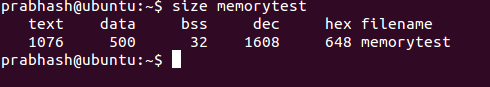`The data segment lost 4bytes of global1 variable.`

`Let us now comment one uninitialized global variable global2.`

`#include <stdio.h>`
`//int` `global1 = 20; ``/* initialized global variable stored in DS*/`
`//int global2;    /* Uninitialized global variables stored in BSS segment*/`
`int global3=0;  /* Global variables initialized to zero are stored in BSS segment*/`
`int` `main(``void``)`
`{`
`    ``static` `int` `i = 100; ``/* Initialized static variable stored in DS*/`
`  static int j=0;    /* Static variables initialized to 0 are stored in BSS segment*/ `
`    //int l=100;  /* stack stores the auto variables*/       `
`    int k; ``     /* stack stores the auto variables*/ `

` ``return` `0;`
`}`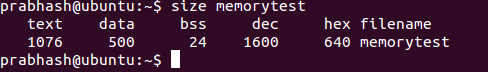As expected the BSS segment lost some data, but 8 bytes? Why so? when I just deleted one int.
`Let us all lots of global variables to see where the variables cluster around in memory.`

` #include <stdio.h>int global10; //1050int global11; //1044int global12;// 104cint global3=0; //1038 /* Global variables initialized to zero are stored in BSS segment*/int global13=10; //1018int global15=20;  //101cint global16=30;  //1020int global17;  //1048int global18=0;  //103c `
`int main(void){    static int i = 100; /* Initialized static variable stored in DS*/  static int j=0;    /* Static variables initialized to 0 are stored in BSS segment*/    //int l=100;  /* stack stores the auto variables*/         int k;      /* stack stores the auto variables*/ `
`return 0;}`

`Let us see the memory map of the global variables used in this process`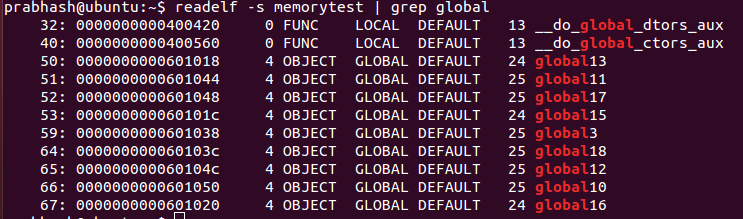` `
We can see that the global variables global11,global17, global3, global18,global12,global10 cluster together towards higher memory address, understandably in the BSS segment whereas global variables global13, global15 and global 16 cluster towards lower address( Data segment).

Let us now check for the address of static variables i and j.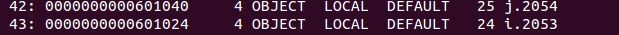`Variable i is at lower address i.e data segment as it is initialized to 100 whereas variable j is at BSS segment(higher address).`

`Keypoints-`
`Local variables get address from stack memory.`
`Global and static initialized variables get memory in data segment.`
`Global and static uninitialized variables get memory in bss segment.`
`Global and static 0 initilized variables are put either in bss or data segment(preferably bss).`
`Heap is used for runtime user allocated memory using malloc.`

` `

` `

#### 1 comment:

1.